# ASHRAE Direct Beam IAM

This model uses the incidence angle modifier coefficients based on the ASHRAE parameterization.

## Inputs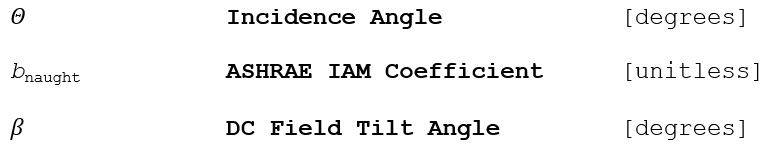## Outputs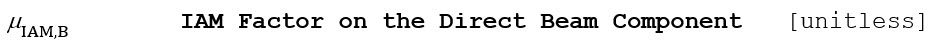## Algorithm (Direct Beam Component)

1.) Compute the direct beam incidence angle attenuation coefficient as a function of the incidence angle.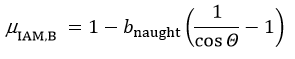2.) The incidence angle function approaches a singularity and is only valid at incidence angles less than 87°.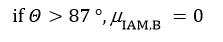3.) Reject all shading factors where the sun is found to be behind the plane of the array, i.e. the back of the module may be lit if the solar incidence angle is less than zero: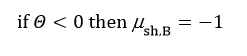The shading factor here is assigned to a value of -1 (in order to distinguish it from 0 (fully shaded) and 1 (unshaded). A negative shading factor does not have any effect on the irradiance and will not contribute to the “shading loss” calculation.